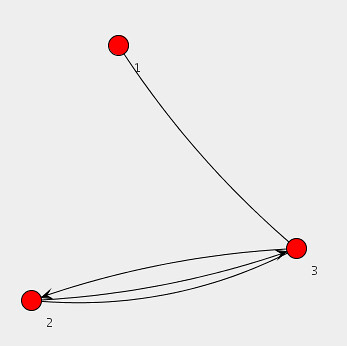# A Brief Summary of Independent Set in Graph Theory

### Graph Basics

Let G be a undirected graph. G=(V,E), where V is a set of vertices and E is a set of edges.  Every edge e in E consists of two vertices in V of G. It is said to connect, join, or link the two vertices (or end points).

### Independent Set

﻿﻿﻿An independent set S is a subset of V in G such that no two vertices in S are adjacent. I suppose that its name is meaning that vertices in an independent set S is independent on a set of edges in a graph G. Like other vertex sets in graph theory, independent set has maximal and maximum sets as follows:

The independent set S is maximal if S is not a proper subset of any independent set of G.

The independent set S is maximum if there is no other independent set has more vertices than S.

That is, a largest maximal independent set is called a maximum independent set. The maximum independent set problem is an NP-hard optimization problem.

All graphs has independent sets. For a graph G having a maximum independent set, the independence number α(G) is determined by the cardinality of a maximum independent set.

### Relations to Dominating Sets

• A dominating set in a graph G is a subset D of V such that every vertex not in D is joined to at least one member of D by some edge.
• In other words, a vertex set D is a dominating set in G if and if only every vertex in a graph G is contained in (or is adjacent to) a vertex in D.
• Every maximal independent set S of vertices in a simple graph G has the property that every vertex of the graph either is contained in S or is adjacent to a vertex in S.
• That is, an independent set is a dominating set if and if only it is a maximal independent set.

### Relations to Graph Coloring

• Independent set problem is related to coloring problem since vertices in an independent set can have the same color.

# Data-Intensive Text Processing with MapReduce Draft Available in Online

Data-Intensive Text Processing with MapReduce, Jimmy Lin and Chris Dyer

Actually, there have never been books that directly deal with MapReduce programming and algorithms. This book addresses from MapReduce algorithm design to EM Algorithms for Text Processing. Although this book is still draft, it seems well-organized and very interesting. In addition, the book contains some basic graph algorithms using MapReduce.

# Java Universal Network/Graph Framework

Recently, I’m primarily concerned with large-scale graph data processing. Occasionally, the visualization of graph can be a good way for us to observe some properties from graph data sets. Today, I’m going to introduce a graph framework, called Java Universal Network/Graph Framework (Jung). Jung provides data structures for graph, a programming interface familiar with graph features, some fundamental graph algorithms (e.g., minimum spanning tree, depth-first search, breath-first search, and dijkstra algorithm), and even visualization methods. Especially, I’m interested in its visualization methods.

The following java source shows the programming interface of Jung. In more detail, this program make a graph, add three vertices to the graph, and connect vertices. This source code is brought from Jung tutorial. As you can see, Jung’s APIs are very easy.

```  // Make a graph by a SparseMultigraph instance.
Graph&lt;Integer, String&gt; g = new SparseMultigraph&lt;Integer, String&gt;();
g.addVertex((Integer)1); // Add a vertex with an integer 1
g.addVertex((Integer)2);
g.addVertex((Integer)3);
g.addEdge(&quot;Edge-A&quot;, 1,3); // Added an edge to connect between 1 and 3 vertices.
g.addEdge(&quot;Edge-B&quot;, 2,3, EdgeType.DIRECTED);
g.addEdge(&quot;Edge-C&quot;, 3, 2, EdgeType.DIRECTED);
g.addEdge(&quot;Edge-P&quot;, 2,3); // A parallel edge

// Make some objects for graph layout and visualization.
Layout&lt;Integer, String&gt; layout = new KKLayout&lt;Integer, String&gt;(g);
BasicVisualizationServer&lt;Integer, String&gt; vv =
new BasicVisualizationServer&lt;Integer, String&gt;(layout);
vv.setPreferredSize(new Dimension(800,800));

// It determine how each vertex with its value is represented in a diagram.
ToStringLabeller&lt;Integer&gt; vertexPaint = new ToStringLabeller&lt;Integer&gt;() {
public String transform(Integer i) {
return &quot;&quot;+i;
}
};

vv.getRenderContext().setVertexLabelTransformer(vertexPaint);

JFrame frame = new JFrame(&quot;Simple Graph View&quot;);
frame.setDefaultCloseOperation(JFrame.EXIT_ON_CLOSE);
frame.getContentPane().add(vv);
frame.pack();
frame.setVisible(true);
```

Some APIs of the Jung are based on generic programming, so you can use easily vertices or edges to contains user-defined data. If you want more detail information, visit http://jung.sourceforge.net.

The above source code shows the following diagram.